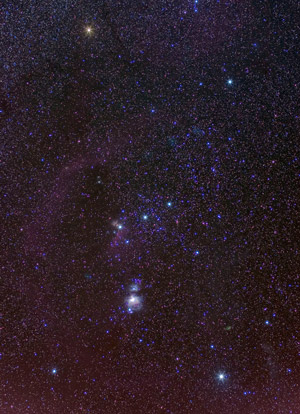# Planck's Law of Black-body Radiation

Interactive plot of Planck's Law

Anyone who's ever used a toaster will have noticed that when the heating elements get hot, they start to emit light. Initially, when they're warming up, the colour is dark red. When they get hotter, they get brighter, and the colour is more orange.

Similarly, next time you're stargazing, take a look at Orion. You might notice that the bottom right star (Rigel), appears bluish, whereas the top left star (Betelgeuse) appears more red.The light emitted by the stars and the toaster's heating elements can be modelled by the black-body radiation curve at the top of the page.

$B_{\lambda}(T) = \frac{2hc^2}{\lambda^5}\frac{1}{e^{\frac{hc}{\lambda k_BT}}-1}$

Where

• $$B$$ is the spectral radiance
• $$T$$ is the absolute temperature of the object
• $$\lambda$$ is the wavelength
• $$h$$ is Planck's constant
• $$c$$ is the speed of light
• $$k_BT$$ is Boltzman's constant

Our eyes can detect radiation that has a wavelength from around 380nm to 740nm. Our eye combines all the intensities in this region together and we perceive this as a colour.

Picture credits: Picture of Orion taken from Wikipedia